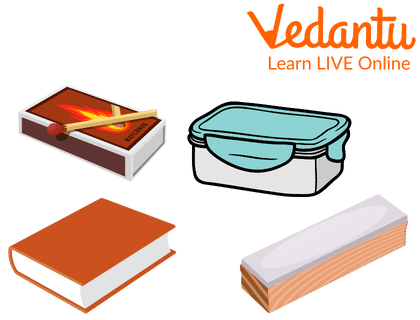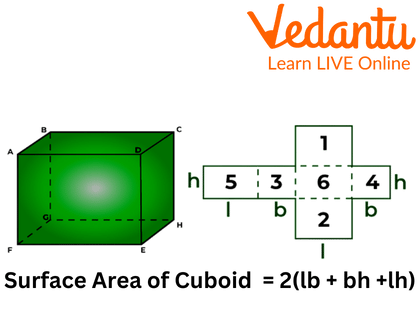Courses
Courses for Kids
Free study material
Free LIVE classes
More

# Volume And Surface Area of Cuboid## What is Cuboid?

A cuboid is around us in day-to-day life. We see it in the form of bricks, shoeboxes, cuboid objects, etc. A cuboid is a three-dimensional figure which has six rectangular faces, twelve edges, and eight vertices. The cuboid shape is formed with a closed rectangular face.

## Cuboid Examples

The boxes we use, the lunch boxes we take to school, the bricks we use to build a house, the pencil box, etc. are the known examples existing around us. These are some common examples that we have seen in our surroundings. The following pictures have some of the common examples.Cuboid Examples

## What is Surface Area?

The surface area is the area of a solid object that measures the surface that occupies the object. The surface area of a cuboid is the area of 6 rectangular faces. There is a formula to calculate the surface area of a cuboid.Surface Area

### Formula for the Surface Area of Cuboid:

Curved surface area : $2h\left( {l + b} \right)$

Total Surface area : $2\left( {lb + bh + hl} \right)$

Where l is length, b is the breadth and h stands for height.

## Cuboid Examples: Surface Area

Example 1: The cuboid has its dimension given as length is 8 cm, width is 6 cm and height is 5 cm. Find the Total surface area of the given cuboid.

Given

Height(h) $= {\rm{ }}5{\rm{ }}cm$

Width(w) $= {\rm{ }}6{\rm{ }}cm$

Length(l) $= {\rm{ }}8{\rm{ }}cm$

Total surface area $= {\rm{ }}2\left( {lb + bh + hl} \right)$

$= {\rm{ }}2\left[ {{\rm{ }}\left( {8 \times 6} \right){\rm{ }} + {\rm{ }}\left( {6 \times 5} \right){\rm{ }} + {\rm{ }}\left( {5 \times 8} \right)} \right]$

$= {\rm{ }}2{\rm{ }}\left[ {{\rm{ }}48{\rm{ }} + {\rm{ }}30{\rm{ }} + {\rm{ }}40{\rm{ }}} \right]$

$= {\rm{ }}2 \times 118$

$= 236$

Hence, the total surface area is $236c{m^2}.$

### Volume Of Cuboid

The volume of a cuboid is the product of length, breadth, and height in cubic units.

The formula of the volume of cuboid: length $\times$ breadth $\times$ height

Let’s understand it by doing some examples.

## Cuboid Example: Volume Of The Cuboid

Example 2: Calculate the volume of a cuboid with a length of 8 cm, breadth of 25 cm, and height of 50 cm.

Given

Length $= \;8{\rm{ }}cm$

Breadth $= {\rm{ }}25{\rm{ }}cm$

Height $= {\rm{ }}50{\rm{ }}cm$

The volume of cuboid: length $\times$ breadth $\times$ height

$= \,8\, \times \,25\, \times \,50$

$= \,10000\,\,c{m^3}$

## Solved Questions:

1. Find the surface area of a cuboid whose length is 5 cm, breadth is 6 cm and height is 9 cm.

Given

Length $= {\rm{ }}5{\rm{ }}cm$

Breadth $= \,6{\rm{ }}cm$

Height ${\rm{ = }}9{\rm{ }}cm$

Surface are of cuboid $= {\rm{ }}2\left( {lb + bh + hl} \right)$

$= {\rm{ }}2{\rm{ }}\left[ {\left( {5 \times 6} \right){\rm{ }} + {\rm{ }}\left( {6 \times 9} \right){\rm{ }} + {\rm{ }}\left( {9 \times 5} \right)} \right]$

$= {\rm{ }}2{\rm{ }}\left[ {{\rm{ }}30 + 54 + 45{\rm{ }}} \right]$

$= {\rm{ }}2 \times 129$

$\; = {\rm{ }}258{\rm{ }}c{m^2}.$

2. Find the volume of the cuboid whose length is 8 cm, breadth is 7 cm and height is 4cm.

Given

Length ${\rm{ = }}8{\rm{ }}cm$

Breadth ${\rm{ = }}7{\rm{ }}cm$

Height ${\rm{ = }}4{\rm{ }}cm$

The volume of the cuboid is length × breadth × height

$= {\rm{ }}8 \times 7 \times 4$

$= {\rm{ }}224{\rm{ }}c{m^3}.$

3. Find the sum of the surface area and the volume of the cuboid whose length is 4 cm, breadth is 2 cm and height is 1 cm.

Given

Length $= {\rm{ }}4{\rm{ }}cm$

Breath $= {\rm{ }}2{\rm{ }}cm$

Height $= {\rm{ }}1{\rm{ }}cm$

Surface area of cuboid $= {\rm{ }}2\left( {lb + bh + hl} \right)$

$= {\rm{ }}2{\rm{ }}\left[ {{\rm{ }}\left( {4 \times 2} \right){\rm{ }} + {\rm{ }}\left( {2 \times 1} \right){\rm{ }} + {\rm{ }}\left( {1 \times 4} \right)} \right]$

$= {\rm{ }}2{\rm{ }}\left[ {{\rm{ }}8{\rm{ }} + {\rm{ }}2{\rm{ }} + {\rm{ }}4{\rm{ }}} \right]$

$= {\rm{ }}2 \times 14$

$= {\rm{ }}28{\rm{ }}c{m^2}$.

The volume of the cuboid is length × breadth × height

$= {\rm{ }}4 \times 2 \times 1$

$= {\rm{ }}8{\rm{ }}c{m^3}.$

Sum of the surface area and the volume $= \,\,28\,\, + \,\,8\,\, = \,\,36\,cm$

## Summary

In this chapter, we have studied the cuboid. A cuboid is a three-dimensional solid object and the measure that the object occupies is the surface of the cuboid and the product of length, breadth, and height is the volume of the object.We also solved the various examples and solved questions to understand the surface area and volume of a cuboid.

Last updated date: 05th Jun 2023
Total views: 47.7k
Views today: 0.42k

## FAQs on Volume And Surface Area of Cuboid

1. Give a real life cuboid example and state why it is a cuboid.

The Brick is a real life Cuboid Example as the brick has 6 Rectangular faces and 12 edges.

2. State any 2 characteristics of the cuboid.

It has 12 edges and it has 12 vertex

3. Can the volume of the cuboid be calculated using the height of the cuboid?

No, We cannot calculate the volume of a cuboid without using the height of the cuboid. We need to find all the three components like length , breadth and height to calculate the Volume of Cuboid.